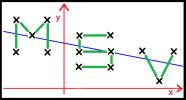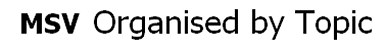AS Level Statistics - S1 Module

1. Exploring Data

MSV 28: World-wide Statistics
MSV 24: Quartiles for a Small Data Set
MSV 4: Outlier Tester

2A.Data Presentation
MSV 1: Histogram Cut-up

2B. Measures of Centre and Spread
MSV 29: Cricketing MMM
MSV 9: Do we divide by n or n-1?
MSV 21: Spot the Error
MSV 34: A Small Sample

3. Probability
MSV 27: Random Independence
MSV 32: Biassed Dice Independence
MSV 23: Balls in a Box
MSV 13: The Colin and Phil Problem
MSV 25: The Independent School
MSV 35: The Two Dominoes

4. Discrete Random Variables
MSV 5: DRVs from a Bag
MSV 37: DRV Venn Diagram
MSV 31: The Four-sided Dice

5. Permutations and Combinations

6. Binomial Distribution
MSV 3: Most Likely Value
MSV 40: The Binomial Mean and Variance
MSV 11: Binomial Reverse

7. Hypothesis Testing using the Binomial Distribution

MSV 12: Significance Levels

A2 Level Statistics - S2 Module

1. The Poisson distribution
MSV 22: Rain
MSV 36: Poisson or not?
MSV 38: Adding Two Poisson Distributions
MSV 30: A Close Approximation

2. The Normal Distribution
MSV 18: The Coffee Problem
MSV 6: Parameter Gaps
MSV 26: Adding Two Normal Distributions

3A. Sampling and Hypothesis Testing using the Normal Distribution
MSV 8: Sampling
MSV 16: Generating Random Numbers
MSV 21: Spot the Error
MSV 14: Sample Mean Gap-Filler

3B. Contingency Tables and the Chi-Squared Distribution
MSV 15: Test Table
MSV 39: Defining the Chi-squared Distribution
MSV 10: Chi-squared and Percentages

4. Bivariate Data, Regression and Correlation
MSV 17: Ten-Point PMCC
MSV 20: Residuals

Other Resources
MSV 2: Markov Chains